# Integration by Substitution JEE Notes | EduRev

## JEE : Integration by Substitution JEE Notes | EduRev

The document Integration by Substitution JEE Notes | EduRev is a part of the JEE Course Mathematics (Maths) Class 12.
All you need of JEE at this link: JEE

C. INTEGRATION BY SUBSTITUTION
Let g be a function whose range is an interval l, and let f be a function that is continuous on l. If g is differentiable on its domain and F is an antiderivative of f on l, then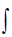f(g(x))g'(x) dx = F(g(x)) + C.
If u = g(x), then du = g'(x) andf(u) du = F(u) + C .

GUIDELINES FOR MAKING A CHANGE OF VARIABLE
1. Choose a substitution u = g(x). Usually, it is best to choose the inner part of a composite function, such as a quantity raised to a power.
2. Compute du = g'(x) dx.
3. Rewrite the integral in terms of the variable u.
4. Evaluate the resulting integral in terms of u.
5. Replace u by g(x) to obtain an antiderivative in terms of x.

THE GENERAL POSER RULE FOR INTEGRATION
If g is a differentiable function of x, then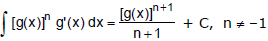RATIONALIZING SUBSTITUTIONS
Some irrational functions can be changed into rational functions by means of appropriate substitutions.
In particular, when an integrand contains an expression of the form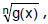then the substitution u =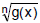may be effective.

SOME STANDARD SUBSTITUTIONS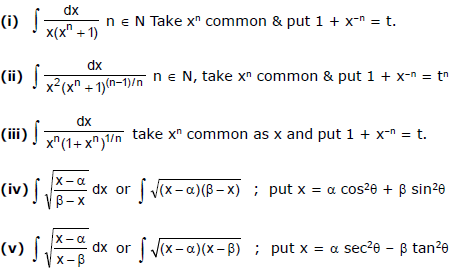Ex.8 Evaluate(x2 +1)2 (2x) dx .
Sol. Letting g(x) = x2 + 1, we obtain g'(x) = 2x and f(g(x)) = [g(x)]2.

From this, we can recognize that the integrand and follows the f(g(x)) g'(x) pattern. Thus, we can write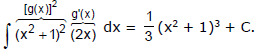Ex.9 Evaluate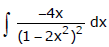Sol.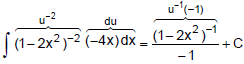Ex.10 Evaluate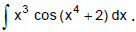Sol.

Let u = x+ 2   ⇒   du = 4x3 dx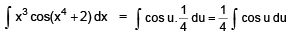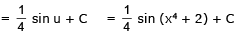Ex.11 Evaluate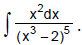Sol.

Let u = x3 – 2. Then du = 3x2 dx. so by substitution :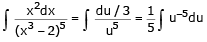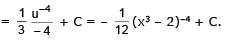Ex.12 Evaluate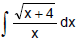Sol. Let u  =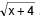. Then u= x + 4, so x = u–4 and dx = 2u du.

Therefore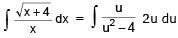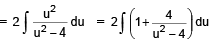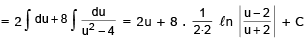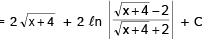Ex.13 Evaluate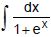Sol. Rewrite the integrand as follows :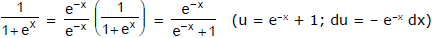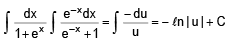= – ln (e-x + 1) + c   (∴  e-x + 1 > 0)

Ex.14 Evaluatesec x dx

Sol. Multiply the integrand sec x by sec x + tan x and divide by the same quantity :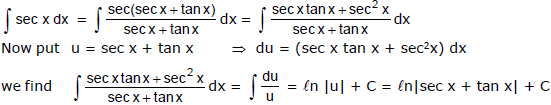Ex.15 Evaluatecos x (4 - sin2 x) dx
Sol. Put sin x = t so that cos x dx = dt. Then the given integral =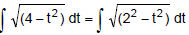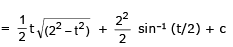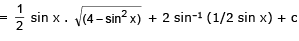Ex.16 Integrate

(i)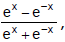(ii)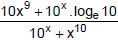Sol.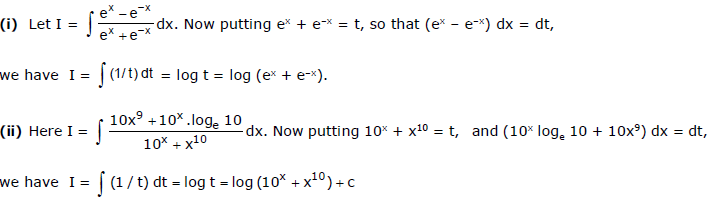Ex.17 Integrate

(i)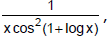(ii)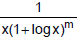Sol.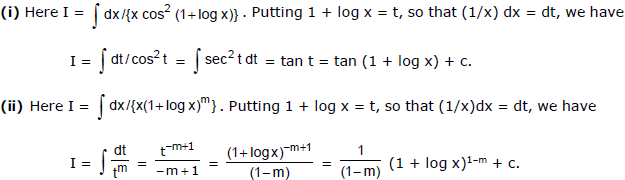Ex.18 Integrate

(i)(ii)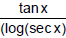Sol.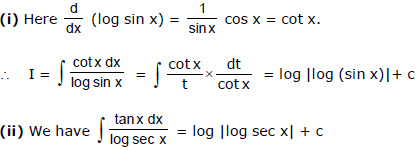Ex.19 Integrate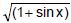Sol.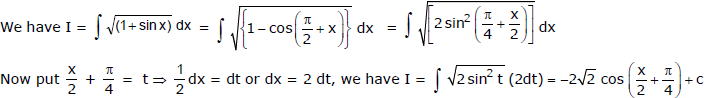Ex.20 Integrate cos5x.

Sol.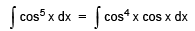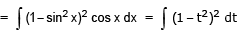[put sin x = t ⇒ cos x dx = dt]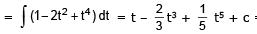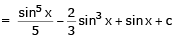Ex.21 Evaluate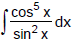Sol.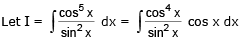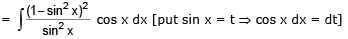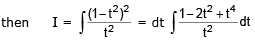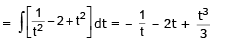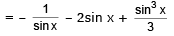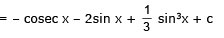Ex.22 Integrate 1/(sin3 x cos5x).

Sol. Here the integrand is sin–3 x cos–5x. It is of type sinm x cosn x,where m + n = –3 –5 = –8 i.e., –ve even integer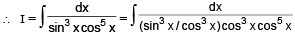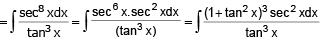Now put tan x = t so that secx dx = dt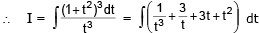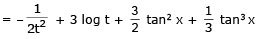Ex.23 Integrate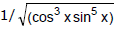Sol. Here the integrand is of the type cosm x sinnx. We have m = –3/2, n = – 5/2, m + n = – 4 i.e., and even negative integer.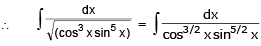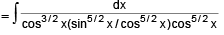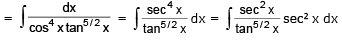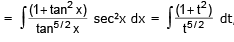,putting tan x = t and sec2x dx = dt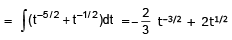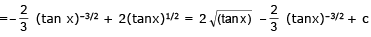Ex.24 Evaluate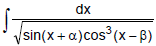Sol.

Put x – β = y  ⇒  dx = dy

Given integral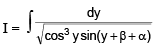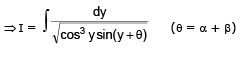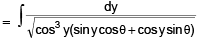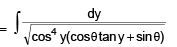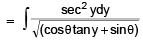Now put sinθ + cosθ tan y = z2 ⇒  cosq sec2 y dy = 2z dz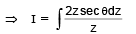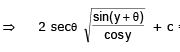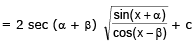Ex.25 Evaluate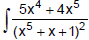dx

Sol.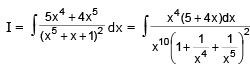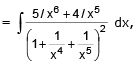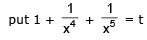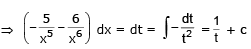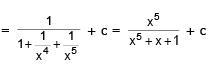Offer running on EduRev: Apply code STAYHOME200 to get INR 200 off on our premium plan EduRev Infinity!

## Mathematics (Maths) Class 12

209 videos|222 docs|124 tests

,

,

,

,

,

,

,

,

,

,

,

,

,

,

,

,

,

,

,

,

,

;